# Chromatic Adaptation Transform by Reflectance ReconstructionPublished October 15, 2018 by Scott Allen Burns, last updated May 8, 2020

Change Log
10/15/18 Initial publication.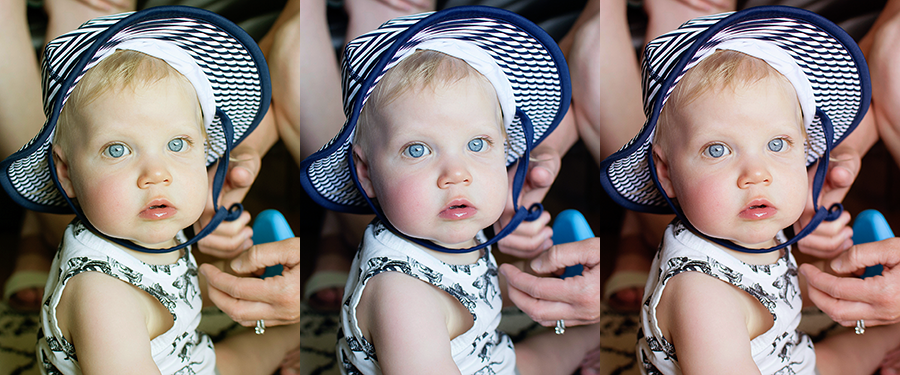##### Preface

This page is an early version of work relating to chromatic adaptation. The topic was eventually developed further and published in preprint form here, and later published in the journal Color Research & Application (Burns SA. Chromatic adaptation transform by spectral reconstruction. Color Res Appl. 2019;44(5):682-693).

This webpage remains online mostly because it provides a “gentle” introduction to what a chromatic adaptation transform is and does.

#### Introduction

The color stimulus that enters our eyes when we view an object can be thought of as the product of an illuminant and an object reflectance. Both are usually described as a distribution over a range of visible wavelengths. The color sensation we experience can be modeled as the product of the stimulus and a matrix of color matching functions (CMFs), suitably scaled to account for the “reference white” of the illuminant. The outcome of this computation is a triplet of values, XYZ, called tristimulus values. These tristimulus values can be further transformed into other color spaces, such as RGB or L*a*b*.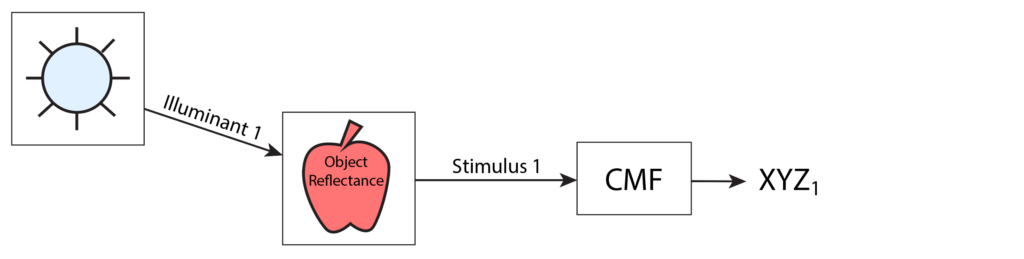When the illuminant changes, it is a simple matter to determine how the tristimulus values will change by recomputing the product of the new illuminant and the object reflectance, giving a new stimulus, and then forming the product of new stimulus and the CMFs to form a new set of tristimulus values.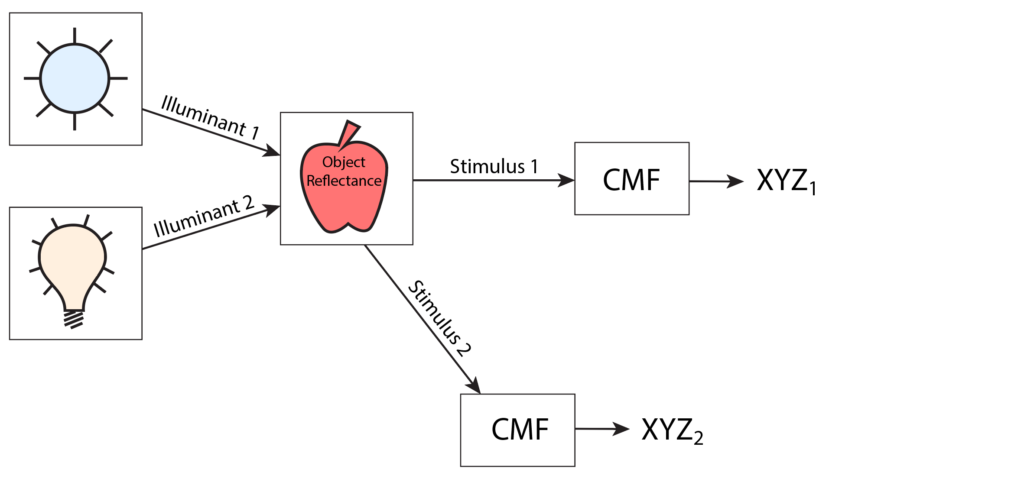Predicting the change in tristimulus values due to a change in illuminant is a common task, such as in image editing programs like Photoshop. In this case, we do not have access to the reflectance properties of the objects being viewed in the image. Instead, we have only three color-space coordinates (e.g., sRGB) at each pixel. This is where the “chromatic adaptation transform” comes into play.

The chromatic adaptation transform (CAT) is a mathematical way to predict how tristimulus values will change when moving from one illuminant environment to another. A well-known form of this transform is the Bradford CAT. It is simply a 3×3 matrix (unique to the source and destination illuminants) that gets multiplied by the source tristimulus values (XYZ1 in the figure) to produce another set of tristimulus values (XYZD in the figure) that approximate what the true tristimulus values would be in the environment of the new illuminant (XYZ2 in the figure). I emphasize the word “approximate” because without the reflectance information of the objects in the scene of the image, there is no way to precisely compute the adapted tristimulus values XYZ2.

The Bradford CAT (as well as other von Kries type CATs, such as CMCCAT2000, CMCCAT97, and CAT02) operate by transforming the tristimulus values to a space of “cone responses,” which are intended to model how the three types of cones in our retina behave. Then these cone response values are scaled according to a function of both the old and new illuminants, and then are transformed back to the original tristimulus space. Nowhere in this process are the reflectance properties of the objects in the image utilized directly. Note that the transform matrix relies only on the tristimulus values of the illuminant white points, not the full illuminant spectra.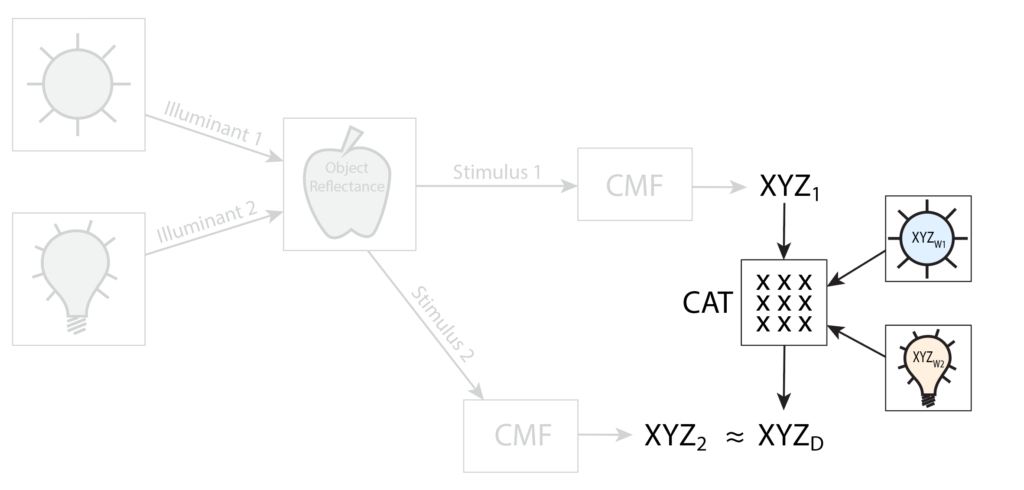In contrast, I am proposing a new approximate way to predict the change in tristimulus values using reconstructed reflectances instead of cone response manipulations. The idea is simple: (1) construct a high-quality reflectance curve approximation from the source tristimulus values and the source illuminant, (2) apply the destination illuminant to the reconstructed reflectance to produce a reconstructed stimulus, (3) compute the destination tristimulus values from the reconstructed stimulus.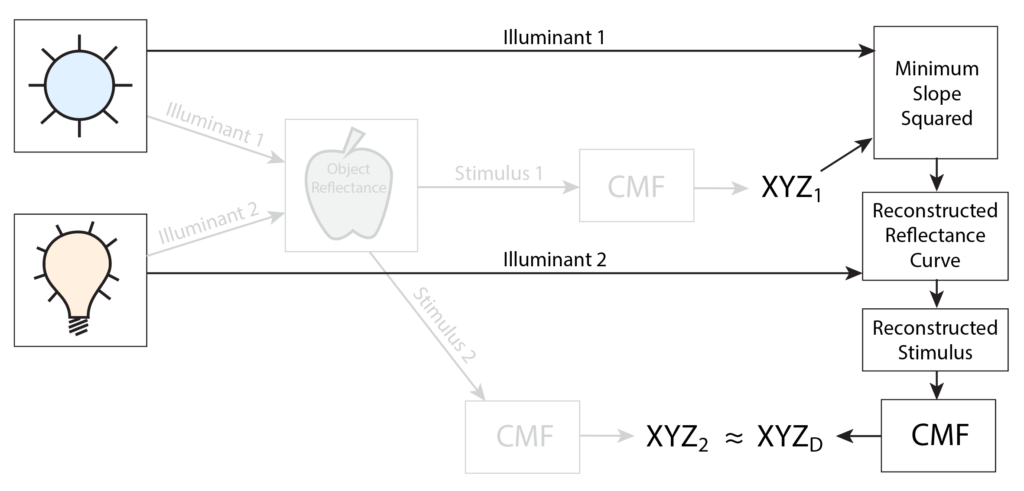#### Reflectance Reconstruction

I published a technique in 2015 for reconstructing reflectance curves corresponding to a given sRGB triplet (link). The idea behind the reconstruction is that the single reflectance curve with the least integral of slope squared (or equivalently, the least RMS of slope of the reflectance curve) will result in a realistic-looking reconstructed reflectance curve. Further investigation revealed that these reconstructed reflectances match surprisingly well with spectrophotometrically-measured reflectances of common colored objects, specifically Munsell Book of Color chips and commercially available paints and pigments.

The optimization statement that produces this least slope squared reflectance is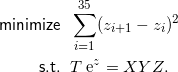The optimization takes place in the space of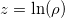, where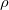is the reflectance curve, discretized over 36 wavelength bands from 380 nm to 730 nm in 10nm intervals. Working in log space eliminates the possibility of the reflectance going negative because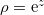is always positive.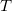is a 3×36 matrix that transforms reflectanceto tristimulus values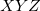, and is computed as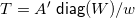. Matrix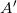is 3×36 and contains the color matching functions by rows.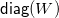is a 36×36 matrix with the illuminant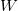on the main diagonal and zeros elsewhere. Scalar valueis the inner (dot) product of the second row ofand. Vectorin the constraint set is the 3×1 target tristimulus triplet. The constraint set,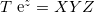, ensures that the reflectance curve and illuminant produce tristimulus values that match the specified.

The solution of the optimization can be achieved several ways. A general-purpose optimization solver can be used, such as Matlab’s fmincon function or Excel’s Solver Add-in. The computational effort is substantial in this case. Instead, it is useful to take advantage of the special structure of this nonlinear program, which is described in detail in the “LLSS” section of the prior publication mentioned above. Here is a Matlab program that solves the optimization in an efficient way using the LLSS method.

To demonstrate the quality of the reconstructed reflectances, the six plots below present six Liquitex-brand acrylic paint colors, showing spectrophotometrically-measured reflectances (blue curves) and the reconstructed reflectances generated by the LLSS algorithm (red curves):

Notice how there tends to be more discrepancy between the original reflectance curve and the reconstructed one at the very high and low wavelengths. Human vision is far less sensitive to these outer wavelengths, so these discrepancies have very little impact on perceived color. The optimization process takes advantage of this reduced visual sensitivity and keeps the slope as close to zero as possible at both ends of the spectral range. Keep in mind that even though the two curves may differ considerably at the ends, they both share the identical tristimulus values and perceived color.

#### Proposed Chromatic Adaptation Transform Algorithm

The proposed CAT algorithm is as follows:

1. Given a source tristimulus triplet, XYZ_S, and a source illuminant, W_S, construct a reflectance curve, rho, using the LLSS_XYZ code.
2. Compute the destination tristimulus triplet, XYZ_D, from the reconstructed stimulus (product of rho and a destination illuminant, W_D).

Here is a three-line Matlab function that does this, using the Matlab LLSS_XYZ function discussed above:

 function XYZ_D = CAT_LLSS(A, XYZ_S, W_S, W_D) rho = LLSS_XYZ(A, W_S, XYZ_S); XYZ_D = A*diag(W_D)*rho/(A(2,:)*W_D); 

If a different color space is desired, such as sRGB, then simply wrap the function above inside transformations to and from XYZ:

 XYZ_S = sRGB_to_XYZ(sRGB_S); XYZ_D = CAT_LLSS(A, XYZ_S, W_S, W_D); sRGB_D = XYZ_to_sRGB(XYZ_D); 

#### Proof of Concept

This section compares the Bradford adaptation method to the new proposed one for a variety of source and destination illuminants. To check the quality of the adapted XYZ values, it is necessary to have reflectance information about the objects being evaluated. Here, we use the Gretag-Macbeth ColorChecker, shown to the right. Measured reflectance properties of each color are available, and this study used the set from the BabelColor website located here. Note that the measured reflectance information was used only to assess the quality of the CAT results, and not used by either method to produce them.

For each of the first 18 colors in the ColorChecker, both the proposed method and the Bradford method was used to adapt the source XYZ values using all combinations of pairs of illuminants A, B, C, D50, D55, D65, D75, and E (equal energy). The color difference between the predicted destination XYZD and the true XYZ computed under the destination illuminant was found using the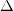ECIE 1976 color difference measure. For each pair of illuminants, the RMSE and maximumE were computed over ColorChecker colors and tabulated.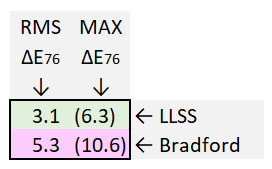In each box of the table, the RMSE is on the left and the maximumE is in parentheses on the right. The green row represents the proposed method results and the pink row represents the Bradford results.The proposed method has roughly half the color difference of Bradford, indicating a much better prediction of how the ColorChecker tristimulus values will respond to a change in illumination.

#### Computational Effort

While the results for the proposed method are very good in comparison to the Bradford CAT, there is considerable computation required. Timing studies show that the computational effort of the adapted XYZ using the new method is about 200 times that of the Bradford computation (which is just a simple matrix multiply). If a CAT is being applied to every pixel of an image, this large computational demand may not be warranted.

To address this, I considered other, less computationally intensive reflectance reconstruction methods. Referring to the previous work cited above, I re-did the study using these two additional methods:

ILSS (Iterative Least Slope Squared)and

LSS (Least Slope Squared)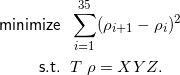ILSS requires about 10 times the computational effort of Bradford. LSS has the same computational effort as Bradford (a simple matrix multiply). The price to be paid for the increased efficiency is that the quality of the reconstructed reflectances (in terms of how well they match colors found in nature) decreases as we move from LLSS to ILSS to LSS.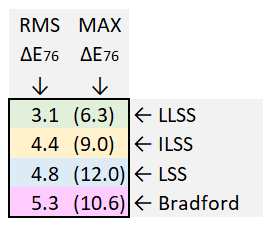Here is a summary of the performance of the four methods. Each box has four rows: green for LLSS, yellow for ILSS, blue for LSS, and pink for Bradford.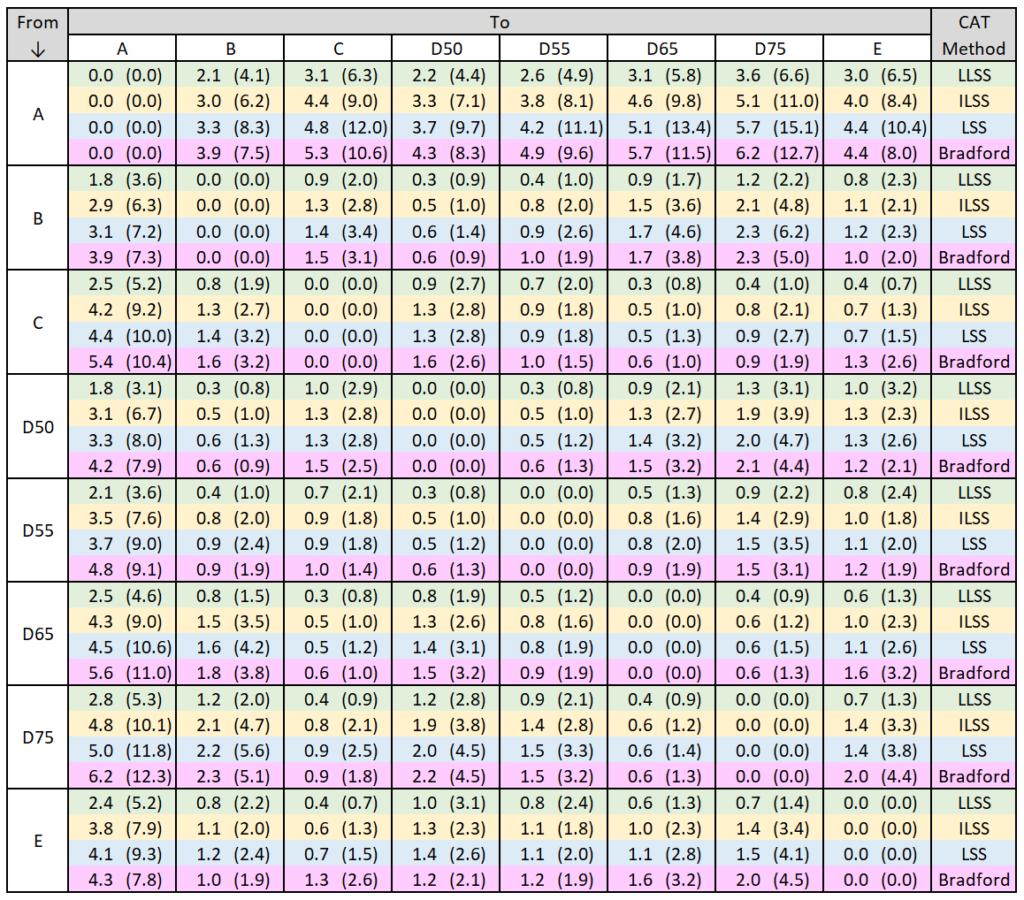ILSS generally performs better than both LSS and Bradford. LSS is comparable to Bradford, having slightly smaller RMS values in general, but occasionally higher maximum values. Since the computational effort for LSS matches that of Bradford, it is a viable candidate for image processing tasks. In the next section we’ll see that in some circumstances, LSS performs much better than Bradford.

#### Extreme Illuminants

For one final comparison study, more extreme illuminants were considered, namely three fluorescent types (standard illuminants F1, F4, and F12), plus three vapor discharge types (metal halide, mercury vapor, and high pressure sodium). These illuminants were obtained from this Excel file. The results are shown below.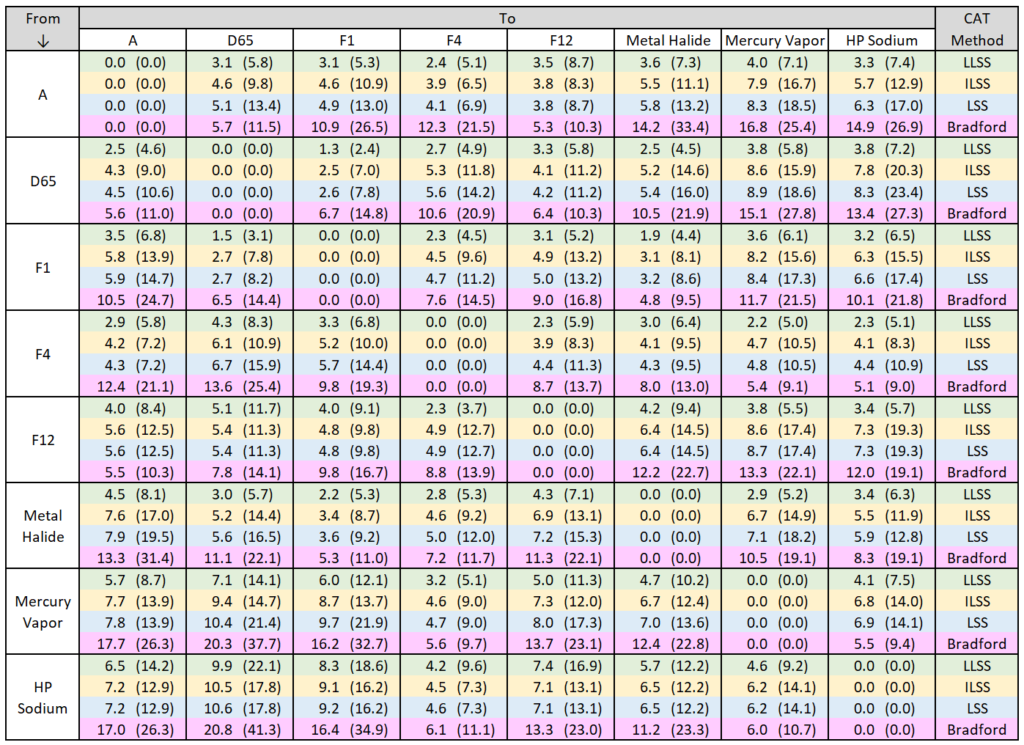This study reveals that the Bradford CAT has difficulty responding to illuminants with spectra that have spikes of energy at various wavelengths. This is not surprising considering that Bradford only knows about the illuminant white point tristimulus values, XYZW, which mask the spikes in the illuminant distribution. Even the least capable of the three proposed methods (LSS) greatly outperforms Bradford when transforming to or from these more extreme conditions.

#### Computing the LSS CAT

To compute the 3×3 CAT matrix for the LSS method, given the source illuminant,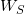, and the destination illuminant,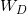, first compute the two matrices that convert reflectance to tristimulus values, referenced to the two illuminants: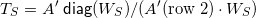and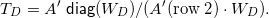Next, find the inverse of the following matrix: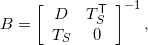where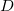is a 36×36 tridiagonal matrix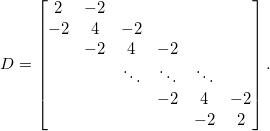Now extract the upper-right 36×3 part of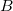, called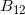. The 3×3 CAT matrix for the LSS method is then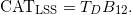Note thatdepends only on the two illuminants and can be computed ahead of time. To apply it to a specific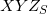, simply perform the matrix multiplication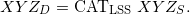#### Future Directions

The Bradford CAT has been superseded in recent years by some newer, more complex CATs, such as CMCCAT2000 and CAT02. Bradford was chosen for this study because of its ease of implementation. It would be useful to extend this study to consider some of these newer CATs as well. Another possible direction for investigation is to cast the spectral distributions in the frequency domain instead of wavelength domain. The slope of the reflectance curve will differ when plotted against frequency, and this may have an impact on the results.

________________________________________

________________________________________